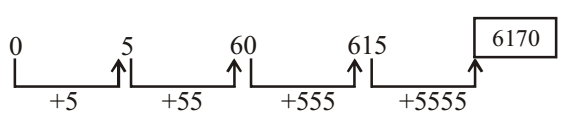## Number Series

#### Number Series

Direction: What will come in the place of question mark (?) in the following number.

1. 0, 5, 60, 615, ?
1. 6030
2. 6170
3. 6130
4. 6000
5. None of these

1. From given figure , it is clear that the increasing order of series given as -
First term = 0
Second term = First term + 5 = 5 ,
Third term = Second term + 55 = 60
Similarly , we can calculate all others terms also.##### Correct Option: B

From given figure , it is clear that the increasing order of series given as -
First term = 0
Second term = First term + 5
Second term = 0 + 5 = 5
Third term = Second term + 55
Third term = 5 + 55 = 60
Similarly , we can find all others terms also.
Fourth term = Third term + 555
Fourth term = 60 + 555 = 615
Fifth term = Fourth term + 5555
Fifth term = 615 + 5555 = ?
Fifth term ( ? ) = 6170